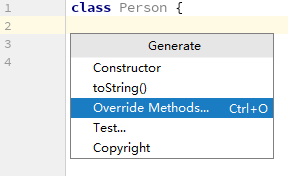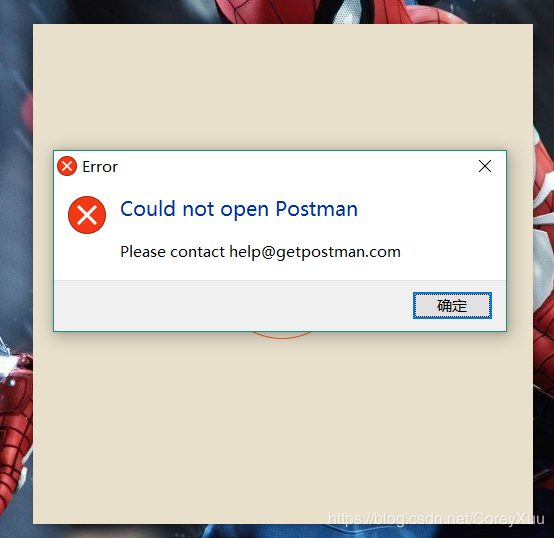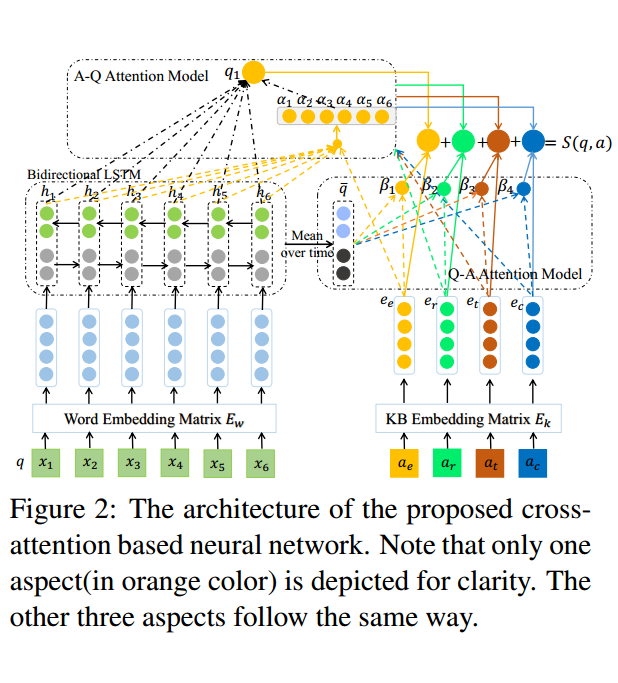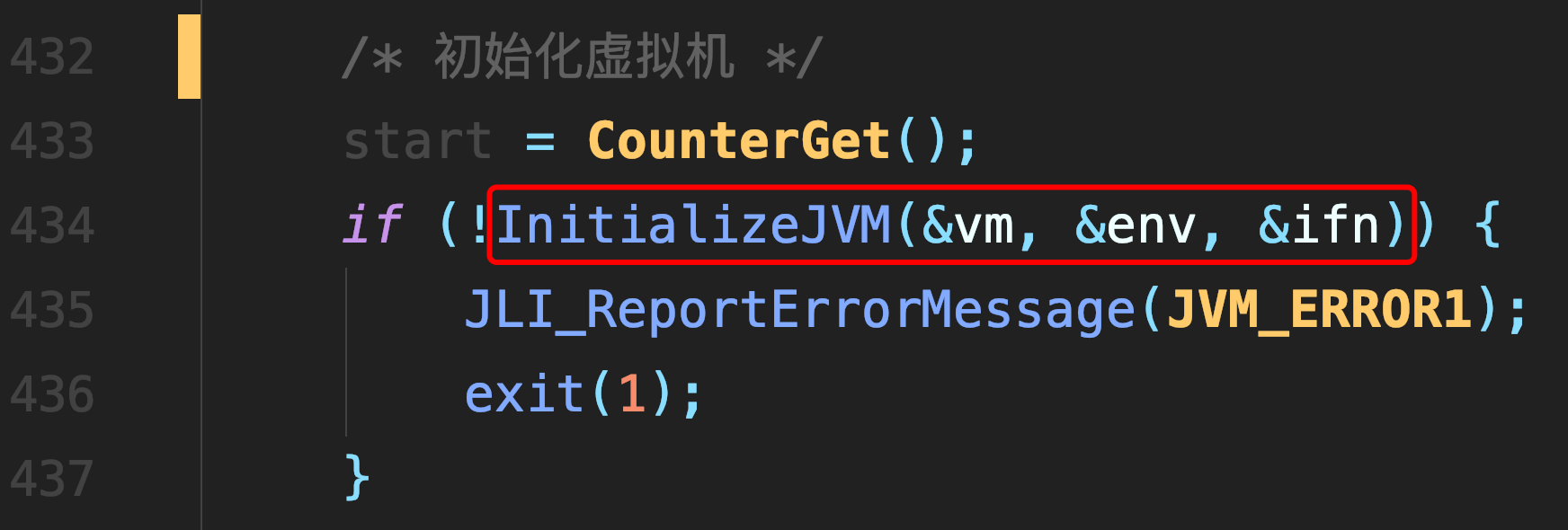# 1116. Come on! Let's C (20)

200 ms

65536 kB

16000 B

Standard

CHEN, Yue

"Let's C" is a popular and fun programming contest hosted by the College of Computer Science and Technology, Zhejiang University. Since the idea of the contest is for fun, the award rules are funny as the following:

0. The Champion will receive a "Mystery Award" (such as a BIG collection of students' research papers...).
1. Those who ranked as a prime number will receive the best award -- the Minions (小黄人)!
2. Everyone else will receive chocolates.

Given the final ranklist and a sequence of contestant ID's, you are supposed to tell the corresponding awards.

Input Specification:

Each input file contains one test case. For each case, the first line gives a positive integer N (<=10000), the total number of contestants. Then N lines of the ranklist follow, each in order gives a contestant's ID (a 4-digit number). After the ranklist, there is a positive integer K followed by K query ID's.

Output Specification:

For each query, print in a line "ID: award" where the award is "Mystery Award", or "Minion", or "Chocolate". If the ID is not in the ranklist, print "Are you kidding?" instead. If the ID has been checked before, print "ID: Checked".

Sample Input:
```6
1111
6666
8888
1234
5555
0001
6
8888
0001
1111
2222
8888
2222
```
Sample Output:
```8888: Minion
0001: Chocolate
1111: Mystery Award
2222: Are you kidding?
8888: Checked
2222: Are you kidding?思路简单的逻辑题，记录下选手排名和是否已经给过奖品就行。注意没在排行榜上的id多次查询后还是输出"Are you kidding?"而不是"Checked"。代码```
```#include<iostream>
#include<cstdio>
#include<map>
#include<math.h>
#include<vector>
using namespace std;

bool isPrime(const int num)
{
if(num == 2)
return true;
if(num % 2 == 0)
return false;
for(int i = 2;i < num;i++)
{
if(num % i == 0)
return false;
}
return true;
}

int main()
{
int N;
while(cin >> N)
{
map<int,bool> dic;
vector<int> rankList(10010);
for(int i = 1;i <= N;i++)
{
int number;
cin >> number;
dic.insert(pair<int,bool>(number,false));
rankList[number] = i ;
}
int K;
cin >> K;
for(int i = 0;i <K;i++)
{
int query;
cin >> query;
printf("%04d",query);
if(dic.count(query) <= 0)
{
cout << ": Are you kidding?" << endl;
continue;
}
if(dic[query])
{
cout << ": Checked" << endl;
continue;
}

if(rankList[query] == 1)
{
dic[query] = true;
cout << ": Mystery Award" << endl;
}
else if(isPrime(rankList[query]))
{
dic[query] = true;
cout << ": Minion" << endl;
}
else
{
dic[query] = true;
cout << ": Chocolate" << endl;
}
}
}
}```

` `

##### 热门推荐
•浏览(655)
•浏览(565)
•浏览(548)
•浏览(488)
•浏览(434)
•浏览(424)
•浏览(381)
•浏览(367)
•浏览(362)
•浏览(358)